This video was brought to you by GeneralPAC.com, making power systems Intuitive, Open and Free for Everyone, Everywhere. Consider subscribing and supporting through patreon.com/GeneralPAC. This is a mechanism for you to support us financially so we can continue making high quality power system video tutorials. Our corporate sponsor for this topic is AllumiaX.com from Seattle, Washington. Contact them for industrial and commercial power system studies.

... a tutorial on the Newton Raphson Power Flow Method, part 4. This example comes from the Grainger and Stevenson's Power System Analysis book that is used across many universities all over the world. This is example 9.4 within this book, so you could look at the book for this example and follow along and learn about the Newton Raphson method. And this is by no means going into the theory of the method but this is more of understanding the Newton Raphson method by example.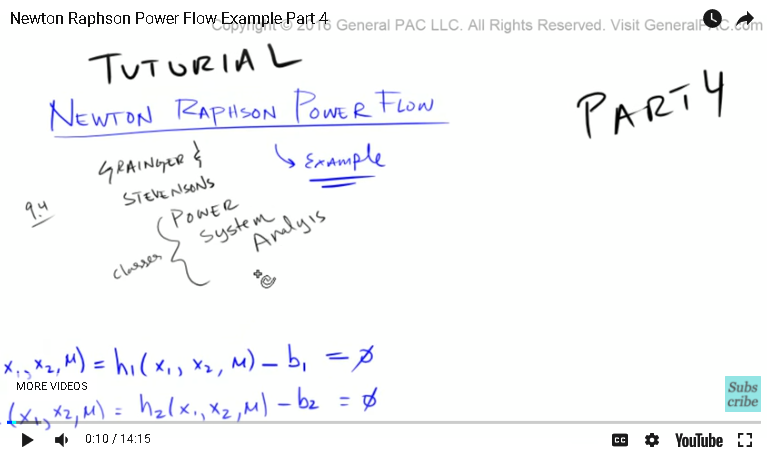So in the previous parts we kind of built up our case and we introduced the formulas and we introduced our Jacobean matrix and we plugged in our initial values to determine the mismatch. We plugged in initial values to determine the Jacobean matrix. And then we calculated the Delta X1 and Delta X2 for the [zeroith 00:00:58] iteration, plugging in our initial values of course, where we have to invert our Jacobean matrix to find out what these guys were.

And then we calculated the values for the second iteration, which are Delta X1 and Delta X2 for the second iteration, right? So now in this part, we're going to go over the second iteration very quickly so we solidify this example.

So in the second iteration, we already know what Delta X1 of 1 is, which is going to equal to negative 0.150 and D X2 for the second iteration is equal to 0.925. Okay?

Now, let's first calculate our mismatch. Remember, our mismatch was simply Delta G1, but now it's for the second iteration. That is equal to B1 minus H1 for the second iteration. The values that we plug in are going to be different, so X1 is this value here. X2 is this value there. And then of course U, we're going to set that to just equal 1. So the values that we plug in is negative 0.150 for X1, 0.925 for X2, and then just 1. So that is equal ... Well, our B1 didn't change, right? B1 was still equal to negative 0.60. So B1 was still equal to negative 0.60, and then B2 was equal to negative 0.30. That didn't change.

So B1 is equal to negative 0.60 and then H1 was going to equal 4UX2 sin of X1, and so this term go to 1. X2 is going to be a little bit different. X2 was this term right here, so that's going to equal 0.925, and then X1 is this term right there, so X1 is equal to negative 0.150. When you evaluate this via a calculator, you have to remember that this term here, that is a term in radians, right? It's not an angle but it's in radians so make sure that the calculator is set to radian.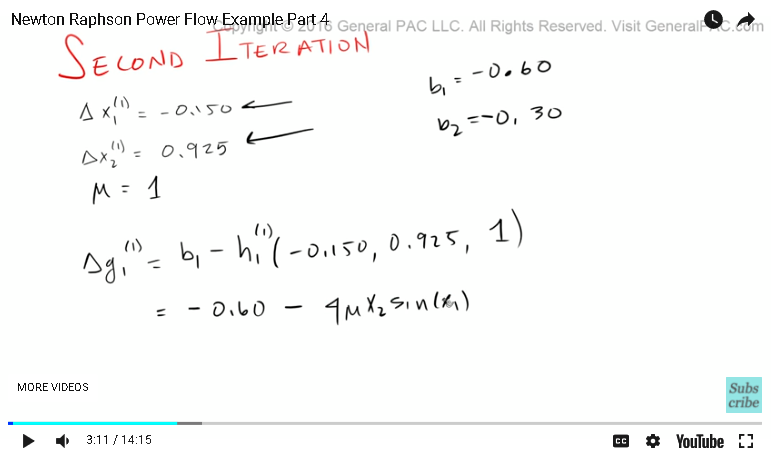So that is going to equal negative 0.60 minus ... it will turn out to be a negative value, 0.5529. Okay? And that my friends is going to equal negative 0.047079. Now, make sure you do this math to keep my honest but that sounds about right. So we're taking negative 0.6 and we're adding .5529. That sounds about right. And this matches the book too, so we know that's right.

So, Delta G2 for the second iteration, that's going to equal again B1 minus H2, and then we're also going to put in our initial values, 5 and then 1, that's going to equal ... remember, B1 was negative 0.30 minus ... now, H2, we have to go back and figure out what that H2 term was, right? So H2 is right there. So that's going to be 4X2 squared minus 4UX2 times cosine of X1 ... was 4X2 squared minus ... I remember this, minus 4UX2, and then I think it was a cosign, the cosign of X1. Yeah, that sounds right.

So again, we have to put in our initial conditions. X2 is this term right there, and this is this term right there, and this is U. So X2 is 0.925, so put in X2 there. U is just 1. X2 is .925, and X1 is negative .150. Well, it turns out that this, my friends, is equal to negative 0.23595 when you evaluate it.

Okay, great. So now we have Delta G1 for the second iteration, and that's going to equal negative 0.047079. Delta G2 for the second iteration is going to equal negative 0.06405. So the next part is determining the partial derivatives. So the partial derivative of DG 1 ...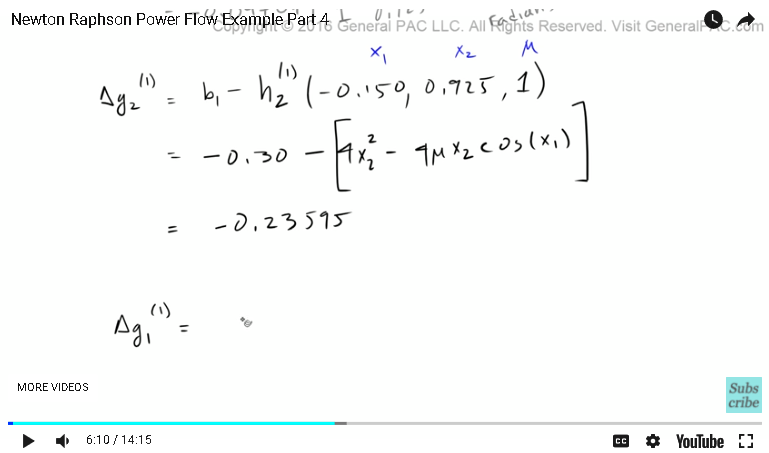Okay, so at this point here, I took a small break and when I came back and resumed, for some reason the video did not get recorded. So I had gone through and marched through all this math and it wasn't recorded. So in this last part of the video, I'll just go through the steps that I have done to complete the second iteration of this example.

So in step one, we calculated the mismatch, Delta G1 and Delta G2, and we had these two values. Now in step two, we have to calculate our Jacobean matrix with the X1 ... with the previous iterated X1 X2 and U values. Remember, in our previous iteration X1, X2 and U ... X1 was equal to negative 0.150. X2 was equal to 0.925, and U, we set that to simply equal 1.

Now, we go through this iteration and remember, this partial differential equation, this equation right here, is equal to 4U times X2 times cosign of X1, and now we just enter in the iterative values in here where U is 1, X2 is .925, and X1 is negative .150, and we get this answer right there.

Similarly for this partial differential equation, we enter in these initial values and then here is a partial differential equation right there. once we enter in the initial values it spits out that solution.

Similarly, we do that same thing for this partial differential equation. It spits out that answer right there. And this partial differential equation, that spits out this answer right there.

So we go through all of our partial differential equations that define the Jacobean, and this is for the first iteration what we get. Now remember, for J of zero, I believe it was pretty straightforward. It was 4-0-0-4. But the next iteration, it got a little more complicated. So, to find Delta X1 and Delta XU, if you remember correctly, so that's step three: calculate Delta X's. If you remember correctly, we had to invert the Jacobean matrix, and then multiply that by our mismatch. So this right here is our mismatch. And that should equal Delta G1 of the first iteration, and Delta G2 of our first iteration of course.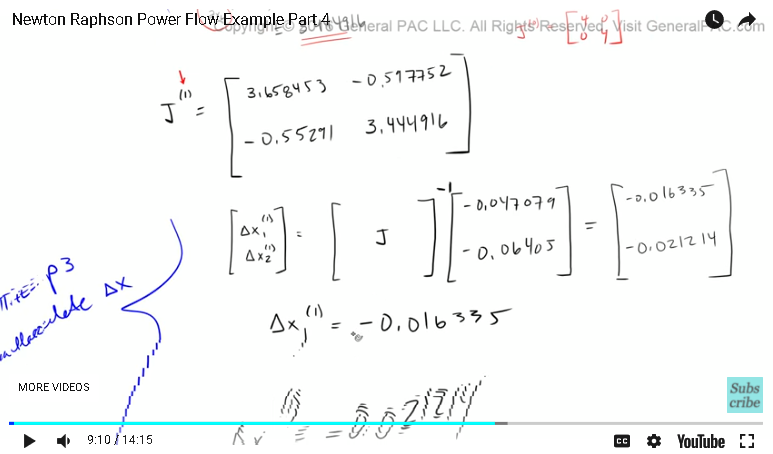So it's the inverse of the Jacobean matrix times our mismatch, and that will equal Delta X1 and Delta X2. Now to confirm that this is in fact our mismatch, we go back over here. So here is where we calculated our mismatch. And sure enough, it is the same so I did in fact copy it correctly.

So once we evaluate that, we get Delta X1 as this value there, and Delta X2 as this value there. So this is the change, right? This is the change in X1 and X2. Okay?

Now, step four is to advance the iteration. Now, X1 of the second iteration, that's going to equal X1 of the first iteration plus the change in X1 of the first iteration. Remember, we said X1 was just negative .150, right? And the change in X1 is going to be this value here. The change in X1 is going to be that value there, and when you subtract both of these values we get negative .16635, which makes sense.

Now, X2 for the second iteration, that's going to equal X2 of the first iteration plus the change in X2 of the first iteration, right? So the first iteration, X2, that was 0.925 plus the change in X2 of the first iteration, that's what we calculated there, so that's going to be entered there, so .925 plus minus .021214, that's going to equal .903786.

And so what we now have ... let me clean this up a little bit. So what we now have is X1 and X2 for the second iteration. Now to solve this problem, or the last step, step five, how do we know when to stop? At what point do we stop iterating our Newton Raphson method? Essentially when we want to stop, the change in X1 and the change in X2 is very very small.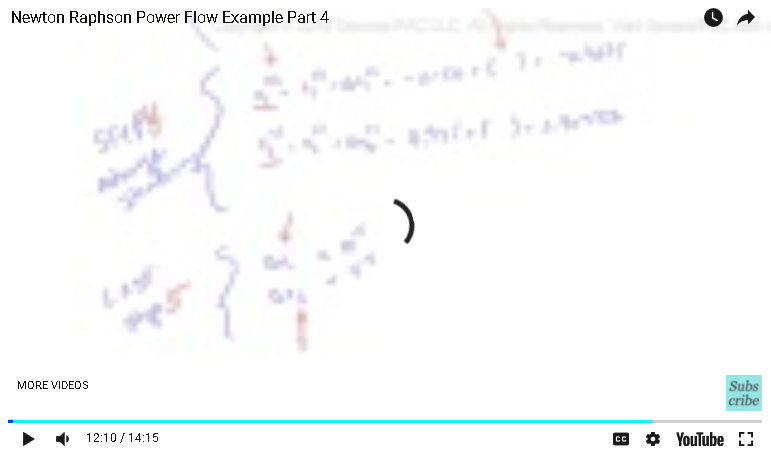So as we're going through this iteration, we will find that the change in X1 and the change in X2, which are these values there, they're going to get smaller and smaller and smaller and smaller, and so we want to stop iterating when these values, the change in X1 and the change in X2, is smaller than some threshold that we set. And in the book, it has set 10 to the negative 5 as that threshold. In the book, they had to go through four iterations. So X1 in the fourth iteration, that in the book ... These are the values that they had given in the book, and once you reach that point, that right there is the solution.

In the book, once you get through the end of this example, they allude this problem to an actual power system. Granted that it's a small power system, it's a [inaudible 00:13:07] system, but this is the procedure that we use to calculate the power flow in a [multi-bus 00:13:16] system. It's much more complicated then that but these are the steps that we use.

Now to conclude this video, if you haven't already please go ahead, click on the bottom right corner of this screen and there's a button to subscribe. Go ahead and subscribe to this video if you found it useful. And of course if you have any questions, there will be a link to a forum where you could sign up and you can ask away about all sorts of questions.

In part five, we're going to take a look in the Power System that the book alluded to when they gave this particular example. So we'll relate these equations to actual values in the Power System and see how they correlate.

Thank you. This video was brought to you by GeneralPac.com, making Power Systems intuitive.

#### Greetings from the GeneralPAC Team!

We make high-quality Power Systems Video Tutorials on complex topics that are free and open to everyone!  Thank you so much for supporting us through Patreon so we can continue doing good and valuable work.

What is Patreon and why do we use it?

Patreon is a fantastic portal that allows our fans and community to make monthly contribution (like Netflix subscription) so we can continue creating high-quality power systems video tutorials. In return, you get access to incredible perks like voting on future topics, getting your questions answered, access to VIP Q/A webinars with the creators of GeneralPAC, and much more! We THANK YOU for supporting us

Why do we need your support?

An incredible amount of time and effort is needed to develop high-quality video tutorials. Each video (Part 1 for example) takes approximately 10 hours to complete which includes learning the concept ourselves, brainstorming creative ways to teach and explain the concepts, writing the script, audio recording, video recording, and editing. It's no wonder why Hundreds-of-Thousands of people have watched, liked, subscribed, and left positive comments on Youtube channel. Your support truly makes all the difference.